21 Major Formula Fails And How To Fix Them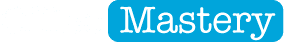21 Major Formula Fails And How To Fix ThemMistakes can creep into your Excel formulas for all sorts of reasons.

In this post, I’m going to walk you through just about every mistake you could make with a formula, starting with the simplest and working through to more complex examples, and show you how to identify, fix and avoid these mistakes in the future.

Feature Download: Grab a printable PDF of everything in this post (completely free). Never forget a key point again!

1. Hashtags in the cell

Kicking things off with something that can throw beginners into a panic. It isn’t really an error as such, but if you don’t recognise what it is, you won’t know how to rectify it.

What am I talking about?

The good old hash symbol, also known as hashes, hashtags, sharps pounds or train tracks.

These symbols will appear if the cell width is not wide enough to display numerical content in full.

How To Fix

Widen the column by positioning the mouse pointer on the right-hand edge of the column letter block, then either double-left-click for a best fit or click and hold the left mouse button then drag the divider to the right to manually resize the column.

2. Wrong construction of formula

Every formula must start with ‘=’ (without the quotes). Starting with ‘+’ also works but is only generally used by those with a Lotus 123 background.

When using functions, every function needs brackets. Some functions have data inside the brackets, e.g.

=SUM(A1, B1, G10:G15)

while others don’t e.g.

=TODAY()

but always make sure you include the brackets.

How To Fix

Every function has the same construct and you must include every part. The construct is

=functionname(arguments)

NB. ‘Arguments’ refers to the data required to be supplied for the function to work. For example, the SUM function needs 1 or more numerical data items or cell references, separated by commas, to work. The VLOOKUP function requires the following arguments:

• the match value
• the data table or array
• the number of the column that contains the result data
• TRUE or FALSE to state whether you need a CLOSEST match or an EXACT match

3. Data without a formula

Some people write formulas like ...

=A1:A10

... thinking that Excel will calculate a total. That’s like saying “Hi Excel, here’s some data” but I'm not going to you what to do with it.

How To Fix

Place the cell range within the brackets of a function, e.g.

4. Not following the BEDMAS rules

There is a set of mathematical rules called the Order of Operations which clearly states the order in which a formula is calculated. The rules have been around since the 1600s and are taught at lower-high-school level.

Here is the order of operations:

B - Brackets

E - Exponential (which means ‘powers’ or ‘to the power of’ like when you square a number)

D - Divide

M - Multiply

S - Subtract

For example, the majority of people say that ...

= 2 + 3 * 4

... is 20, when in fact the answer is 14.

The reason?

The multiplication (3*4) is done first giving an answer of 12. The addition (2+12) is done second, giving an answer of 14.

By adding brackets around the 2+3 like this …

= (2 + 3) * 4

… the answer is now 20, because the bit in the brackets is now performed first, giving an answer of 5. The multiplication - five times four - is now done second, resulting in 20.

Let’s look at another example.

Let’s say that cell A1 contains today’s date and cell A2 contains your date of birth.

As a quick aside, dates in Excel are stored as numbers.

Day 1 is 1-Jan-1900

​Day 2 is 2-Jan-1900

Day 3 is 3-Jan-1900 and so on.

This makes calculations with dates a doddle.

To calculate your age, you can start with today’s date (A1), subtract your date of birth (A2) and divide the result by the number of days in a year (365.25).

Your initial formula will look like this:

= A1 - A2 / 365.25

But if you leave it like this, the formula calculates out of sequence because the division will occur before the subtraction. Therefore you would modify the formula to

= (A1 - A2) / 365.25

P.S. There are a bunch of alternative acronyms for BEDMAS. These include BODMAS, BOMDAS and PEDMAS. They all serve the same purpose. The ‘P’ stands for Parantheses. The ‘O’ stands for Of (i.e. to the power OF), Over, Order or Ordinals.

How To Fix

Be aware of the order of operations. If in doubt, add brackets to be sure your formula calculates in the order you want it to.

5. Not enough brackets, too many brackets or closing brackets are in the wrong position

Whenever you type an opening bracket in a formula you must include a corresponding closing bracket.

This is true whether you are using brackets to control the order of operations or brackets for one or more functions.

Remember to treat every function (in a formula) as a unit. Every function needs a set of brackets.

Where you have nested functions, i.e. one function sitting inside the brackets of another function, the formula calculates from the inside to the outside.

For example,

=IF(AND(A1="QLD", A2>500), "Yes", "No")

The inner brackets belong to the AND function, so the AND function is processed as a unit. The answer is then used as the condition for the IF function, which owns the outer set of brackets.

When editing the formula in Excel, the outer brackets (IF) are black and the inner brackets (AND) are green.

Whenever multiple brackets are used in a formula, they are colour-coded. The outermost brackets are always black, the next inner brackets are always green, the next set are purple and each subsequent inner set of brackets will have its own colour.

This is a very handy way of identifying matching pairs of brackets and should be used to make sure that brackets are placed in the correct positions.

How To Fix

Use the bracket colours to ensure that each opening bracket has a corresponding closing bracket and that brackets are positioned correctly.

6. Typing cell references directly into a formula

I’ll state it outright. You should never type a cell reference directly into a formula.

Why?

Because you could misread it and you could mistype it. Very easy to do.

How To Fix

Once you have started your formula, use the mouse and click on the cells or cell ranges you want to use. Excel will place these references into your formulas without any mistakes caused by human error.

7. Clicking a random cell to finish a formula

When you finish writing a formula you must press ENTER to lock it in. If you don't you could end up with a random cell reference in your formula, which has no right to be there.

Let’s say, you write this formula.

= A1 + A2 + A3

Then, instead of pressing Enter to lock in the formula, you click on the other side of the spreadsheets to get away from the formula. If the cell you click randomly is, say, M5, then the formula now changes to:

= A1 + A2 + M5

And the worst part is, you probably don't notice until later!

How To Fix

Always press Enter to lock in a formula before moving on to the next thing.

8. Incorrect notation

For example, to sum a cell range you would type:

=SUM(A1:B10)

which uses the colon (:) to indicate a cell range. The following alternatives will fail or give incorrect answers:

How To Fix

Use the mouse to select the cell range. Excel will always insert the correct notation.

9. A missing comma

If you forget to separate a data item or a function argument with a comma, it will not work.

Example #1:

=VLOOKUP(A1 B3:G15, 2, FALSE)

fails because of a missing comma between A1 and B3:G15.

Example #2:

=SUM(A1, B2 E3:G10)

fails because of a missing comma between B2 and E3:G10.

How To Fix

Always separate cells, cell ranges or other items of data with a comma. Always separate each argument within a function using a comma.

10. A missing quote

Any formula that includes a text-based value must place quotation marks (") around the text item. If you don’t, the function won’t work.

For example:

= IF (A1="QLD", "Go the maroons", "Go the blues")

works great, but miss off any of the 6 quotation marks and the formula will fall flat.

How To Fix

Make sure you add quotation marks around text items in a formula.

11. A missing argument

As a minimum you must provide all the mandatory data that a function requires to produce an answer, separating each argument or data item with a comma.

For example, VLOOKUP has 3 mandatory arguments (the match value, the data table or array, the number of the column that contains the result data) and 1 optional argument (the range lookup which is TRUE or FALSE depending on whether you need a CLOSEST match or an EXACT match).

If you do not include one or more of the first 3 arguments, the formula will fail.

How To Fix

Check the help documentation and make sure you include every mandatory argument.

Another approach is to click within the function brackets then click the fx icon next to the formula bar. The function box is displayed.

This will provide a box for each argument and it is an easier way to enter your data and understand how a function hangs together.

12. Not conforming to the requirements of the function being used

Sticking with VLOOKUP, the first column of the table, which is used to match the input value, must contain unique entries. If there are any duplicates in the first column, the formula may return the correct result, or it may not, making it as useful as a chocolate teapot.

Obviously, with over 450 functions in the Excel library, I cannot possibly go through every function, pointing out the things you must conform to, but Microsoft provide a very comprehensive description in the Help files.

How To Fix

Make sure you understand any restrictions or pre-requisites for any function you use.

13. #REF! error

A #REF! or reference error occurs when a formula refers to a non-existent cell, caused by physically deleting the column or row where the cell existed.

How To Fix

If you delete a row or column and immediately notice the appearance of #REF! errors, click the UNDO icon (or press Ctrl Z) and reconsider your actions. If you find the #REF! errors hours or days after the column or row was deleted, your only option is to figure out the purpose of the formula and replace the #REF! occurrence with the correct cell reference. Sorry to be the bearer of bad news!

14. #DIV/0! error

This is the simplest of all error to fix. It occurs when the cell that is being divided by is blank or contains zero.

How To Fix

Put a value in the dividing cell or use IFERROR (explained shortly) to hide the error.

15. #NAME! error

There are 2 reasons why you get a #NAME! error.

• You have mis-typed a function name, e.g. =SU(A1:A10) instead of =SUM(A1:A10).
• You have used a non-existent name in your formula.

When you name a cell or cell range using the name box, make sure to eliminate spaces (use underscore instead) and make sure you press Enter to register the name.

When using a name in a formula make sure not to mis-spell it. You’re actually better off just pressing F3 while inside your formula and selecting the name from the list of named cells or ranges that are displayed.

How To Fix

Check your function names to make sure they are spelt correctly. Check that the name used in your formula exists and is spelt correctly.

16. #VALUE! error

A #VALUE! error normally occurs when you feed text into a numerical function.

For example, if your SUM formula includes a text cell, an error will occur. Often, a label (i.e. text) will be listed alongside a numerical value and the label is mistakenly picked up instead of the value.

If a function points to a cell expecting to find numerical data but instead finds text, the formula will fail.

How To Fix

Check the cells referred to in your formula. Make sure that you are not pointing to text.

17. #N/A! error

This error mostly occurs in LOOKUP functions. If the lookup function cannot find a match based on the input value provided, a #N/A! error is shown.

How To Fix

Ensure there is a match for your input value in the data table or array. You can use IFERROR (explained shortly) to hide a #N/A! error if it occurs.

18. #NUM! error

This error occurs if you supply an invalid number to a function argument. For example, you may inadvertently provide a negative number or add a dollar sign (\$) or percent symbol (%) where none is needed.

How To Fix

Remove any extraneous symbols or clutter - just enter the plain number in your formula.

19. #NULL! error

When you refer to cell range (e.g. A1:A10) if you omit the colon (:) and leave a space, the formula will result in a #NULL! error.

20. Circular reference

This is another one where once you understand what is happening, you can fix it quickly.

A circular reference occurs when the cell in which you are writing the formula contains a reference to itself.

For example, if you write a formula in cell A1 that says

=SUM(A1:A5)

then you will get a circular reference.

How To Fix

Check the cell or cell ranges you have used in your formula. One of them will be the cell you are in. Change it.

21. Incorrectly referring to a cell in another worksheet or workbook

When you refer to a cell on the same worksheet, say A1, the cell reference just says A1.

When you refer to a cell on another worksheet, say A1 on a sheet called February, the cell reference now looks like February!A1.

When you refer to a cell on another workbook, say A1 on a sheet called February in a workbook called Finance 2016, the cell reference now looks like [‘Finance 2016’]February!\$A\$1.

When you create this type of formula, you should switch to the other workbook if necessary, click the desired worksheet tab, then select the cell or cell range. Excel will insert the cell reference into the formula using the correct notation.

If you try to type out the full cell reference yourself, there are too many opportunities for you to stuff up. Just don’t do it!

How To Fix

Check the formula.

1.       Make sure there are brackets around the workbook name.

2.      Make sure workbook names that contain more than one word are encapsulated with quote marks.

3.      Ensure workbook names and worksheet names are spelt correctly.

4.      Ensure there is an exclamation mark (!) between the worksheet name and the cell reference.1.

If this all looks good, select the Data tab and click the Edit Links icon. This show all other workbooks that this workbook links to. If the workbook being linked to has moved or been renamed, click Change Source and point to the workbook to re-establish the link.

To round off this post I thought I’d add a few ​Tools & Techniques You Can Use To Identify, Track & Resolve Formula Errors

1. Use the small green triangles and Smart Tags

Sometimes you’ll see a small green triangle in the corner of a cell. This simply indicates that there’s something weird happening in your cell. Common causes are that the cell is inconsistent will all those that surround it, or that a number is stored as text. There are other reasons too.

Click the cell that has the triangle, to display a Smart Tag.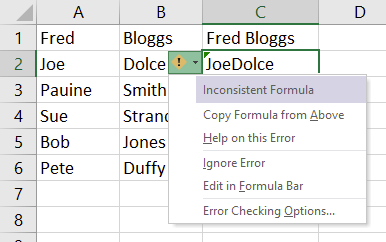Figure 01: Green triangle indicates inconsistent formula

Click the smart tag to see the reason, then choose one of the options presented to take the appropriate action. A screen tip is also displayed which displays the reason for the error.

When you click the smart tag a list of actions is offered. For example:

• You can choose to ignore the error (which will remove the triangle).
• You can get some help on the error (via Microsoft online help).
• You can show the calculation steps to pinpoint where the error is occurring.

2. Use IS and ERROR.TYPE functions to detect and hide particular errors

The IS functions check for a particular type of error within a cell and return TRUE or FALSE.

The structure for all IS functions is =functionname(cell reference or formula).

• ISTEXT - returns TRUE if text
• ISNONTEXT - returns TRUE if not text (e.g. numeric, logical, error etc.)
• ISBLANK - returns TRUE if empty
• ISNUMBER - returns TRUE if numeric
• ISREF - returns TRUE for a cell reference
• ISLOGICAL returns TRUE if a logical value found (i.e. TRUE or FALSE)
• ISERROR - returns TRUE on any error (#N/A, #VALUE!, #REF!, #DIV/0!, #NUM!, #NAME?, or #NULL!)
• ISERR - returns TRUE for any error except #N/AISNA - returns TRUE only for #N/A

The ERROR.TYPE function is a single function which will return a number between 1 and 8 depending on the type of error found.

• 1 = #NULL!
• 2 = #DIV/0!
• 3 = #VALUE!
• 4 = #REF!
• 5 = #NAME?
• 6 = #NUM!
• 7 = #N/A
• 8 = #GETTING_DATA

For example:

=IF(ISNA(VLOOKUP(A1, Lookup_table, 2, FALSE), “”, VLOOKUP(A1, Lookup_table, 2, FALSE)

would show the result of the VLOOKUP function if one was found, otherwise it displays a blank cell (instead of the #N/A! error) if no match is found.

Yes, it's a bit clunky. The next method is much better.

3. Use the IFERROR function

The IFERROR was only introduced in the last few years but simplifies things immensely.

We could simplify the formula in the last step to

=IFERROR(VLOOKUP(A1, Lookup_table, 2, FALSE), "")

If the VLOOKUP does not find a match or returns any kind of error, display nothing instead of displaying the error.

4. Use the Evaluate Formula tool

Let’s consider this simple (if contrived) example.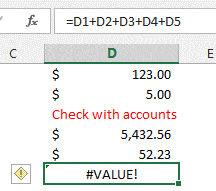Figure 02: A scenario where the Evaluate Formula feature is handy

The formula in cell D6 is manually adding the contents of cells D1 to D5 but cell D3 contains a text entry, which cannot be added.

1.       Select the Formulas tab. Click the Evaluate Formula icon (in the Formula Auditing group). This tool lets you step through the formula one step at a time. The current step is underlined.

2.      Click Evaluate to calculate the result for the underlined step.

The current step is calculated and the next step in underlined.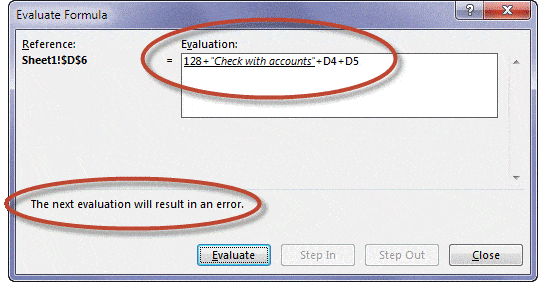Figure 03: The Evaluate Formula dialog box

In this example, processing the current step results in a #VALUE! because it is attempting to add a text item to a numeric item.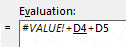Figure 04: The Evaluate step

5. Trace the flow of your data, visually

There is a tool called Trace Precedents which lets you see what data flows into a cell. There is a sister tool called Trace Dependents which lets you see where a cell is used elsewhere.

Both paint arrows on your screen so you can visually see the flow.

To see the precedent cells related to a selected cell:

1.       Click the Formulas tab and click the Trace Precedents icon (in the Formula Auditing group). A blue arrow connects the precedent cell(s) with the selected cell.

2.      Click again to display the cells that are precedent to each of the precedent cells.

3.      Click again to go another level back.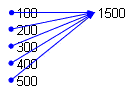Figure 05: Trace Precedents

To display cells that are dependent on the selected cell:

1.       Click the Formulas tab and click the Trace Dependents icon (in the Formula Auditing group). A blue arrow connects the selected cell with all cells dependent on it.

2.      Click again to display cells that are dependent on these dependent cells.

3.      Click again to go another level deeper.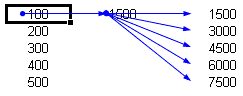Figure 06: Trace Dependents

Let’s Wrap!

In this post I have highlighted 21 different mistakes you can make in your formula and have demonstrated how to rectify each one. I’ve also given you 5 fantastic tools to identify, check for and fix errors.

I trust this has been useful. Now go fix those pesky errors!

Did this clear something up for you? If so, please share on your socials and/or post a brief comment below.

Likewise, if something didn't make sense or you have a question, pop it into the comments below. I'll be waiting ...

Cheers. Here's to your learning and success. Enjoy the rest of your day.FREE CHECKLIST

Download a printable PDF ﻿of this post

PLUS, GET A FREE COPY OF MY 30 EXCEL POWER TIPS